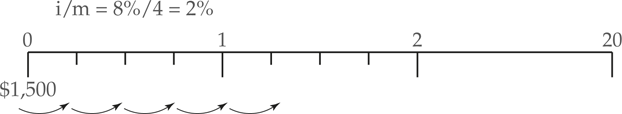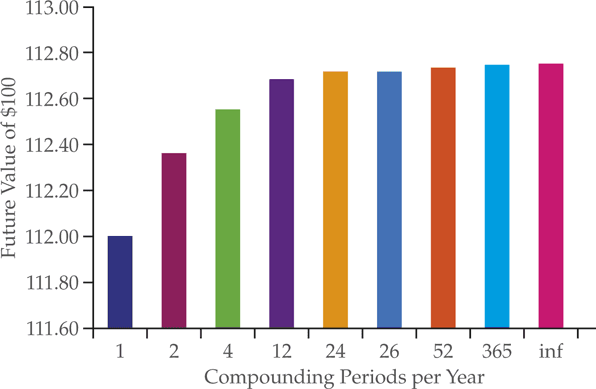# Future Value of Sum with Non-Annual Compounding

Annual compounding refers to a situation in which interest is paid once per year. It is common for interest to be paid more frequently than once per year. We must adjust our formula to allow for any interest payment schedule that may arise.

To calculate a future value with non-annual compounding:

• First, state m as the number of compounding periods in the year. For instance, with semi-annual compounding, m=2.
• Second, match the interest rate to the length of the compounding period. Let’s say there are m compounding periods, then the periodic rate for each period would be i/m. For instance, if the interest rate is 12% per year, then the semi-annual periodic rate is 6%, the quarterly rate is 3%, and the monthly rate is 1%.
• Third, adjust the number of periods by multiplying the number of years with the number of compounding periods per year. For instance, 5 years (n=5) with semi-annual compounding (m=2) is 10 periods (n×m).

The following formula adjusts the equation for future value by inserting the periodic interest rate and the number of compounding periods.

$F{{V}_{n}}=P{{V}_{0}}\times {{\left( 1+\frac{i}{m} \right)}^{n\times m}}$

Example 1 Multiple Compounding Periods per Year

What is the future value of a 1,500 deposit after 20 years with an annual interest rate of 8% compounded quarterly? SolutionIn this example, we know that $\begin{array}{*{35}{l}} i=0.08 \\ m=4 \\ PV=\1,500\\n=20\\\end{array}$ First, solve for the periodic rate: $\frac{i}{m}=\frac{8}{4}=2%$ Second, substitute these numbers into the formula: \begin{align}& F{{V}_{n}}=P{{V}_{0}}\times {{\left( 1+\frac{i}{m} \right)}^{n\times m}} \\& F{{V}_{20}}=\1,500\times{{(1.02)}^{80}}=\7,313.16\\\end{align} Whereas, the future value with annual compounding would be: $F{{V}_{n}}=P{{V}_{0}}\times {{\left( 1+i \right)}^{n}}=1500\times {{\left( 1.08 \right)}^{20}}=\6991.44$ The future value of a1,500 deposit after 20 years at a rate of 8% compounded quarterly is $7,313.16. In Example 1, we found that$1,500 deposited for 20 years at 8% compounded quarterly is $7,313.16. However, the future value with annual compounding is only$6,991.44. It turns out that the more frequently interest is paid, the greater is the future value.